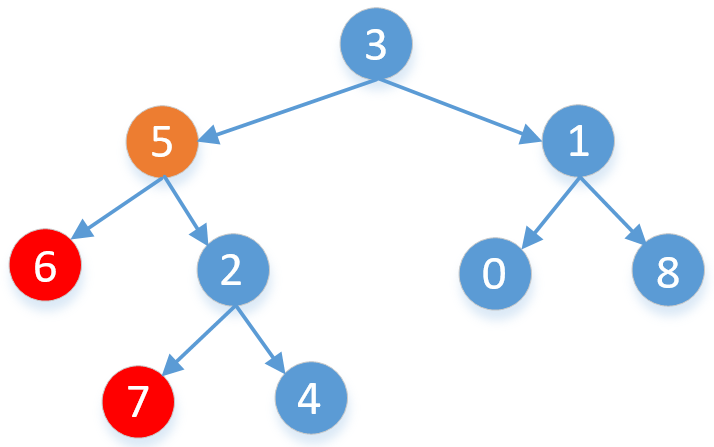public TreeNode lowestCommonAncestor(TreeNode root, TreeNode p, TreeNode q) {
//记录遍历到的每个节点的父节点。
Map<TreeNode, TreeNode> parent = new HashMap<>();
parent.put(root, null);//根节点没有父节点，所以为空
//直到两个节点都找到为止。
while (!parent.containsKey(p) || !parent.containsKey(q)) {
//队列是一边进一边出，这里poll方法是出队，
TreeNode node = queue.poll();
if (node.left != null) {
//左子节点不为空，记录下他的父节点
parent.put(node.left, node);
//左子节点不为空，把它加入到队列中
}
//右节点同上
if (node.right != null) {
parent.put(node.right, node);
}
}
Set<TreeNode> ancestors = new HashSet<>();
//记录下p和他的祖先节点，从p节点开始一直到根节点。
while (p != null) {
p = parent.get(p);
}
//查看p和他的祖先节点是否包含q节点，如果不包含再看是否包含q的父节点……
while (!ancestors.contains(q))
q = parent.get(q);
return q;
}


### 2，递归写法

    public TreeNode lowestCommonAncestor(TreeNode cur, TreeNode p, TreeNode q) {
if (cur == null || cur == p || cur == q)
return cur;
TreeNode left = lowestCommonAncestor(cur.left, p, q);
TreeNode right = lowestCommonAncestor(cur.right, p, q);
//如果left为空，说明这两个节点在cur结点的右子树上，我们只需要返回右子树查找的结果即可
if (left == null)
return right;
//同上
if (right == null)
return left;
//如果left和right都不为空，说明这两个节点一个在cur的左子树上一个在cur的右子树上，
//我们只需要返回cur结点即可。
return cur;
}


#### 如果觉得有用就给个赞吧，还可以关注我的LeetCode主页查看更多的详细题解

8

class Solution {
public TreeNode lowestCommonAncestor(TreeNode root, TreeNode p, TreeNode q) {
if(root==p||root==q||root==null)return root;

TreeNode left=lowestCommonAncestor(root.left, p, q);
TreeNode right=lowestCommonAncestor(root.right, p, q);

if(left==null&&right==null)return null;
if(left!=null&&right!=null)return root;

return left==null ? right:left;
}
}

8/**
* Definition for a binary tree node.
* public class TreeNode {
*     int val;
*     TreeNode left;
*     TreeNode right;
*     TreeNode(int x) { val = x; }
* }
*/
class Solution {
/**
* 节点p和节点q只有两种关系:父子关系 兄弟关系
* 父子关系:
*       1.节点p是节点q的子孙节点,即节点p出现在节点q的左或右子树中;返回q即可;
*       2.节点q是节点p的子孙节点,即节点q出现在节点p的左或右子树中;返回p即可;
* 兄弟关系:
*       节点p,q分别出现在某节点的左子树或右子树中;返回该节点即可;
*
*/
public TreeNode lowestCommonAncestor(TreeNode root, TreeNode p, TreeNode q) {
if(root==null||root==p||root==q)return root;

TreeNode leftRes=lowestCommonAncestor(root.left,p,q);
TreeNode rightRes=lowestCommonAncestor(root.right,p,q);
//p,q在某节点左子树和右子树中
if(leftRes!=null&&rightRes!=null)return root;
//左子树和右子树均没有p,q且也不会该节点
if(leftRes==null&&rightRes==null)return null;

return leftRes==null?rightRes:leftRes;

}
}

3

class Solution {
public:
TreeNode* lowestCommonAncestor(TreeNode* root, TreeNode* p, TreeNode* q) {
if(root==NULL)
{
return root;//空的找个屁，既然有那肯定是根
}
if(root==p||root==q)
{
return root;//既然肯定有，而且有一个就是根，那么直接返回根，
}
//如果上面都不是，那么肯定在左右两边
//根节点往下一层挪，直到根两个中一个相等为止
TreeNode* left=lowestCommonAncestor(root->left,p,q);
TreeNode* right=lowestCommonAncestor(root->right,p,q);
//有三种情况，如果同在左边，那么肯定left不是空，右边为空
//如果同在右边，那么right不是空，左边为空
//如果两边都不为空。那么肯定是根节点
if(left&&right) return root;
return left? left:right;

}
};


1、假设q、p的最近公共祖先是m（m不等于q、p）,则q、p的所有祖先都是m的祖先（m也是m的祖先）。由此可知q、p在m的左右子树。q、p同时在其他祖先（除去m）的左或者右子树上。找到一个左右子树分别有q、p的只能是m。因此if(left!=null&&right!=null)return root;
2、假设q、p的最近公共祖先是q或者p。则进行left？left：right以及root==null||root==p||root==q可得到q或者p。

def lowestCommonAncestor(self, root: 'TreeNode', p: 'TreeNode', q: 'TreeNode') -> 'TreeNode':
# 自顶向下，从root开始，root为空；root为p或者q；root既不是p也不是q，那么转向root的左右子树在其中找p\q
if not root or root ==p or root==q:
return root
l = self.lowestCommonAncestor(root.left, p,q)  # 在左子树中找
r = self.lowestCommonAncestor(root.right, p,q)  # 在右子树中找
if l and r:  # 左右子树都有结果，2节点分列两边，返回父root
return root
if not l and not r:  # 都没找到返回空
return None
return l if l else r  # 只有1个有结果，在同一子树上，返回即可